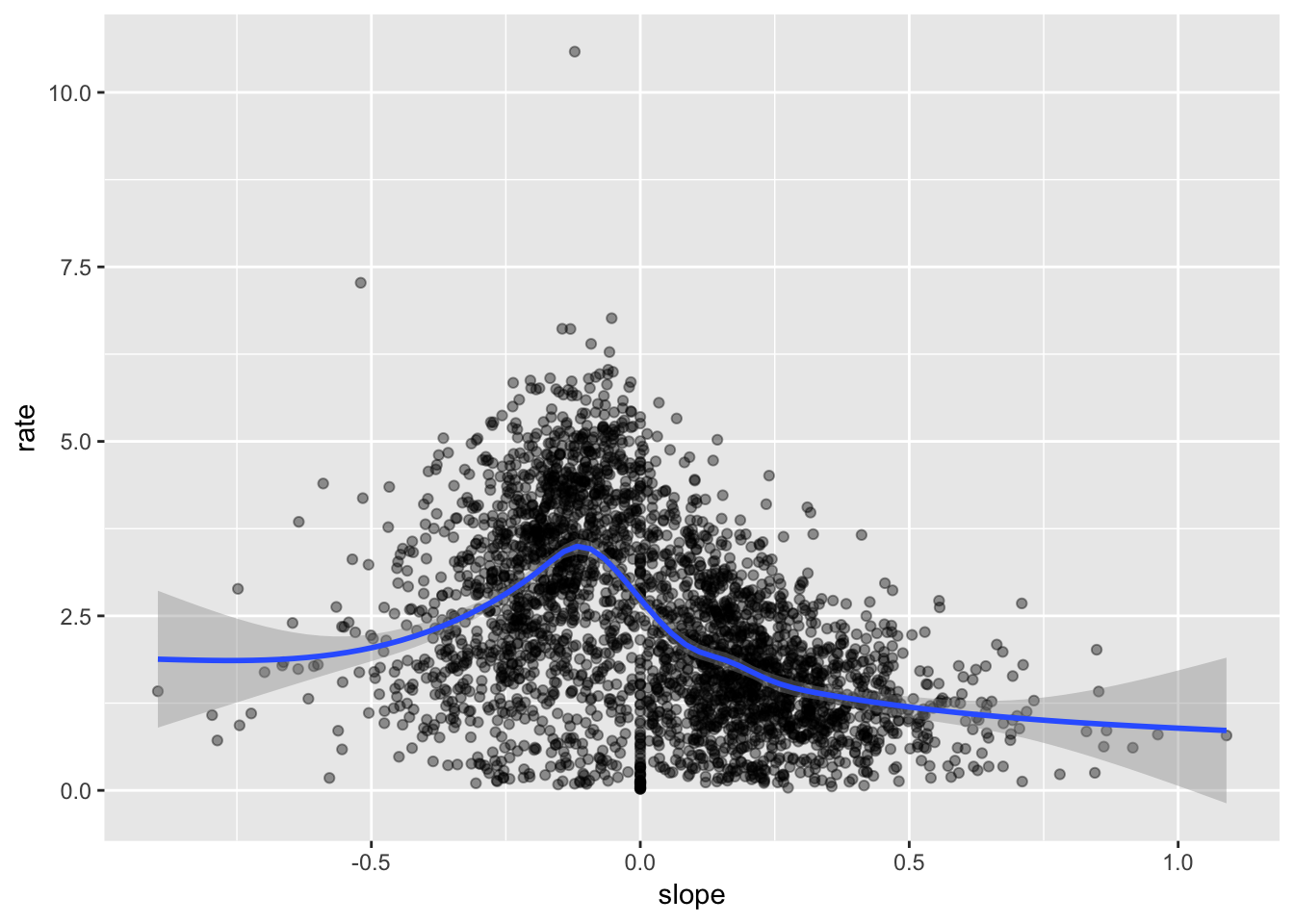# Introduction

Tobler’s hiking function is an exponential function determining the hiking speed, taking into account the gradient. It was formulated by Waldo Tobler. This function was estimated from empirical data of Eduard Imhof and takes the form

$r = 6 \exp\left(-3.5 \left| S + 0.05 \right|\right)$

where

• $$r$$ is the rate of walking (in $$\textrm{kmh}^{-1}$$)
• $$S$$ is the gradient (change in elevation per unit distance walked)
• $$\left| x \right| = \left\{ \begin{array}{rl} x & \textrm{ if x > 0} \\ -x & \textrm{ otherwise} \end{array} \right.$$

The source data is some Strave-generated GPX files relating to various hikes - I have downloaded several of these into a directory called gpx inside my working directory. The files are:

1. Berenthanti_Ghorepani_Ghandruk_Loop_Hike_Day_1_of_3_.gpx
2. Berenthanti_Ghorepani_Ghandruk_Loop_Hike_Day_2_of_3_.gpx
3. Berenthanti_Ghorepani_Ghandruk_Loop_Hike_Day_3_of_3_.gpx
4. Colorado_Belford_Oxford_and_Missouri_Mountains_Hike.gpx
5. Colorado_Longs_Peak_and_Chasm_Lake_Hike.gpx

The code below reads in all of the files in the gpx directory to give a list of data frames, one for each file. Each data frame is then given an id number, and then all of the data frames are merged. The time variable is transformed to the standard lubridate time format, and the elevation is converted to numeric (for some reason it reads in as character). Finally this data frame is converted into a spatial sf object.

files <- dir('gpx') %>% paste0("gpx/",.)
imap(~ .x %>% mutate(id=.y)) %>%
bind_rows() %>%
mutate(time=ymd_hms(time),ele=as.numeric(ele)) %>%
st_as_sf(coords=c('lon','lat'),crs=4326)

The Colorado data is in track ids 4 and 5. Pull out track 4 to start with. To prepare for the analysis, set the projection to EPSG 26954 (appropriate for Colorado) and set the time zone to Mountain Time (as a default, GPX files record time as GMT). Finally, extract the coordinates and add them as ordinary numerical columns called x and y - these will be needed to compute the slope and walking rate.

colorado <- tracks %>% filter(id == 4) %>% st_transform(26954)
mutate(x=xy[,1],y=xy[,2],time=with_tz(time,"America/Denver")) 

Now compute the slope and speed for each point on the track:

colorado <- colorado %>% mutate(tlag = as.numeric(as.period(time - lag(time)),'seconds'),
dist = sqrt((x - lag(x))^2 + (y - lag(y))^2),
rate = 3.6 * dist/tlag,
elag = ele - lag(ele),
slope = elag/dist)

We can now plot the slope against the walking rate. Here a spline smooth is also fitted. Although there is a lot of scatter around the trend, Tobler’s general idea appears sound - on average the rate peaks a a small downward slope, and falls off gradually for increasing or decreasing slope with respect to this reference slope.

colorado %>% ggplot(aes(x=slope,y=rate)) + geom_point(alpha=0.4) + geom_smooth()We can also fit parametric curves - here a Tobler-style hiking function of the form

$r = a \exp\left( -b\left|S + c\right| \right)$

is fitted where:

• $$r,S$$ are as in the original Hiking function
• $$a,b,c$$ are parameters in the hiking function to be estimated.

In Toblers original model, we have $$a=6,b=3.5,c=0.05$$ but here we estimate these parameters in terms of least squares fit, via the R function nls (non-linear least squares).

mod1 <- nls(rate ~ a*exp(-b*abs(slope+c)),
start=list(a=3.6,b=3,c=0.2),
control=nls.control(tol=1.0e-4))
summary(mod1)
##
## Formula: rate ~ a * exp(-b * abs(slope + c))
##
## Parameters:
##   Estimate Std. Error t value Pr(>|t|)
## a 3.565782   0.044255   80.57   <2e-16 ***
## b 2.032773   0.068779   29.55   <2e-16 ***
## c 0.133212   0.004466   29.83   <2e-16 ***
## ---
## Signif. codes:  0 '***' 0.001 '**' 0.01 '*' 0.05 '.' 0.1 ' ' 1
##
## Residual standard error: 1.16 on 3117 degrees of freedom
##
## Number of iterations to convergence: 10
## Achieved convergence tolerance: 9.42e-05
##   (1 observation deleted due to missingness)

A curve can then be created and superimposed on the emprical data to see the quality of the fit.

fitcurve1 <- data_frame(slope=seq(-0.75,0.75,l=101)) %>%
{mutate(.,rate=predict(mod1,.))}
geom_line(data=fitcurve1,col='dodgerblue')